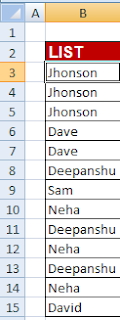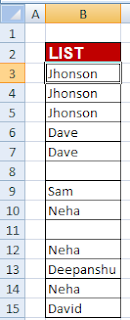# Excel : Count Unique values in a column

This tutorial explains how to count unique values in a column.

Sample DataSample Data
Formula
=SUMPRODUCT(1/COUNTIF(B3:B15,B3:B15))
Logic
The text "Jhonson" appears 3 times so the unique value would be equal to  (1/3) + (1/3) + (1/3) = 1

How Formula works

COUNTIF counts the number of times each value appears.COUNTIF Formula Evaluation
Then all the values are divided by 1 and SUMPRODUCT sums all the fraction values.

Count Unique Values (Ignoring Blank Cells)Data with Blank cells
Formula (Ignoring Blank cells)
=SUMPRODUCT((B3:B15<>"")/COUNTIF(B3:B15,B3:B15&""))

Related Articles
1. 3 Ways to extract unique values
2. Count Unique values in multiple columns
3. Select and Count Duplicate values in ExcelShare
Related Posts